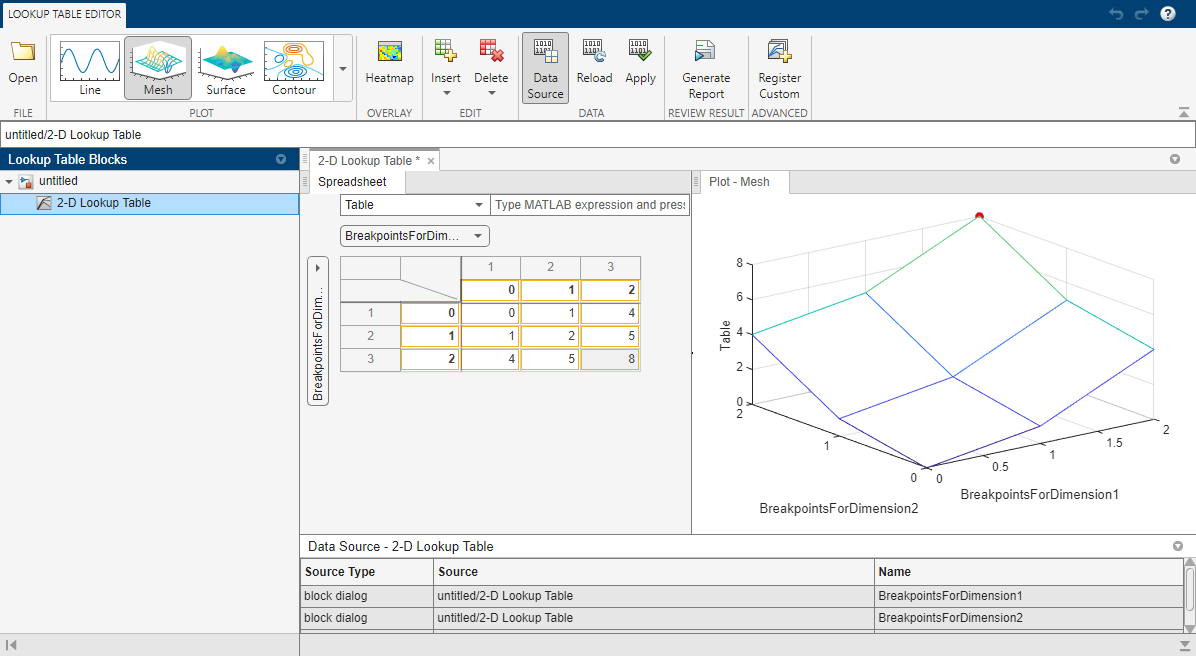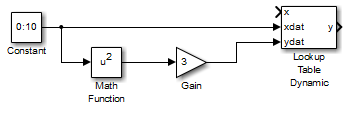## Enter Breakpoints and Table Data

### Entering Data in a Block Parameter Dialog Box

This example shows how to populate a 1-D Lookup Table block using the parameter dialog box. The lookup table in this example approximates the function y = x3 over the range `[-3, 3]`.

1. Copy a 1-D Lookup Table block from the Lookup Tables block library to a Simulink® model.

2. In the 1-D Lookup Table block dialog box, enter the table dimensions and table data in the specified fields of the dialog box:

• Set Number of table dimensions to `1`.

• Set Table data to ```[-27 -8 -1 0 1 8 27]```.

Alternatively, to use an existing lookup table (`Simulink.LookupTable`) object, select Data specification > Lookup table object.

3. Enter the breakpoint data set using either of two methods:

• To specify evenly spaced data points, set Breakpoint specification to ```Even spacing```. Set First point to `-3` and Spacing to `1`. The block calculates the number of evenly spaced breakpoints based on the table data.

• To specify breakpoint data explicitly, set Breakpoint specification to `Explicit values` and set Breakpoints 1 to `[-3:3]`.

### Entering Data in the Lookup Table Editor

Use the following procedure to populate a 2-D Lookup Table block using the Lookup Table Editor. In this example, the lookup table approximates the function z = x2 + y2 over the input ranges `x = [0, 2]` and `y = [0, 2]`.

1. Copy a 2-D Lookup Table block from the Lookup Tables block library to a Simulink model.

2. Open the Lookup Table Editor by selecting Lookup Table Editor from the Modeling tab or by clicking on the dialog box of the 2-D Lookup Table block.

3. Under Viewing "n-D Lookup Table" block data, enter the breakpoint data sets and table data in the appropriate cells. To change data, click a cell, enter the new value, and press Enter.

• In the cells associated with the Row Breakpoints, enter each of the values ```[0 1 2]```.

• In the cells associated with the Column Breakpoints, enter each of the values ```[0 1 2]```.

• In the table data cells, enter the values in the array ```[0 1 4; 1 2 5; 4 5 8]```.

The Lookup Table Editor looks like this:4. In the Lookup Table Editor, select File > Update Block Data to update the data in the 2-D Lookup Table block.

5. Close the Lookup Table Editor.

### Entering Data Using Inports of the Lookup Table Dynamic Block

Use the following procedure to populate a Lookup Table Dynamic block using that block's inports. In this example, the lookup table approximates the function y = 3x2 over the range `[0, 10]`.

1. Copy a Lookup Table Dynamic block from the Lookup Tables block library to a Simulink model.

2. Copy the blocks needed to implement the equation y = 3x2 to the Simulink model:

• One Constant block to define the input range, from the Sources library

• One Math Function block to square the input range, from the Math Operations library

• One Gain block to multiply the signal by 3, also from the Math Operations library

3. Assign the following parameter values to the Constant, Math Function, and Gain blocks using their dialog boxes:

BlockParameterValue
ConstantConstant value`0:10`
Math FunctionFunction`square`
GainGain`3`
4. Input the breakpoint data set to the Lookup Table Dynamic block by connecting the output port of the Constant block to the input port of the Lookup Table Dynamic block labeled xdat. This signal is the input breakpoint data set for x.

5. Input the table data to the Lookup Table Dynamic block by branching the output signal from the Constant block and connecting it to the Math Function block. Then connect the Math Function block to the Gain block. Finally, connect the Gain block to the input port of the Lookup Table Dynamic block labeled ydat. This signal is the table data for y.